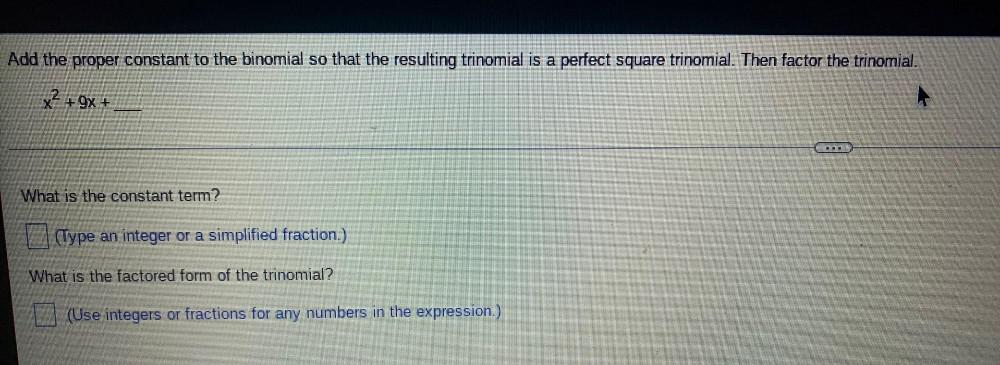Question:

# Add the proper constant to the binomial so that the resulting trinomial is a perfect square trinomial. Then factor the trinomial. x^2 + 9x +______ a)What is the constant term? b)What is the factored fAdd the proper constant to the binomial so that the resulting trinomial is a perfect square trinomial. Then factor the trinomial. x^2 + 9x +______ a)What is the constant term? b)What is the factored form of the trinomial?